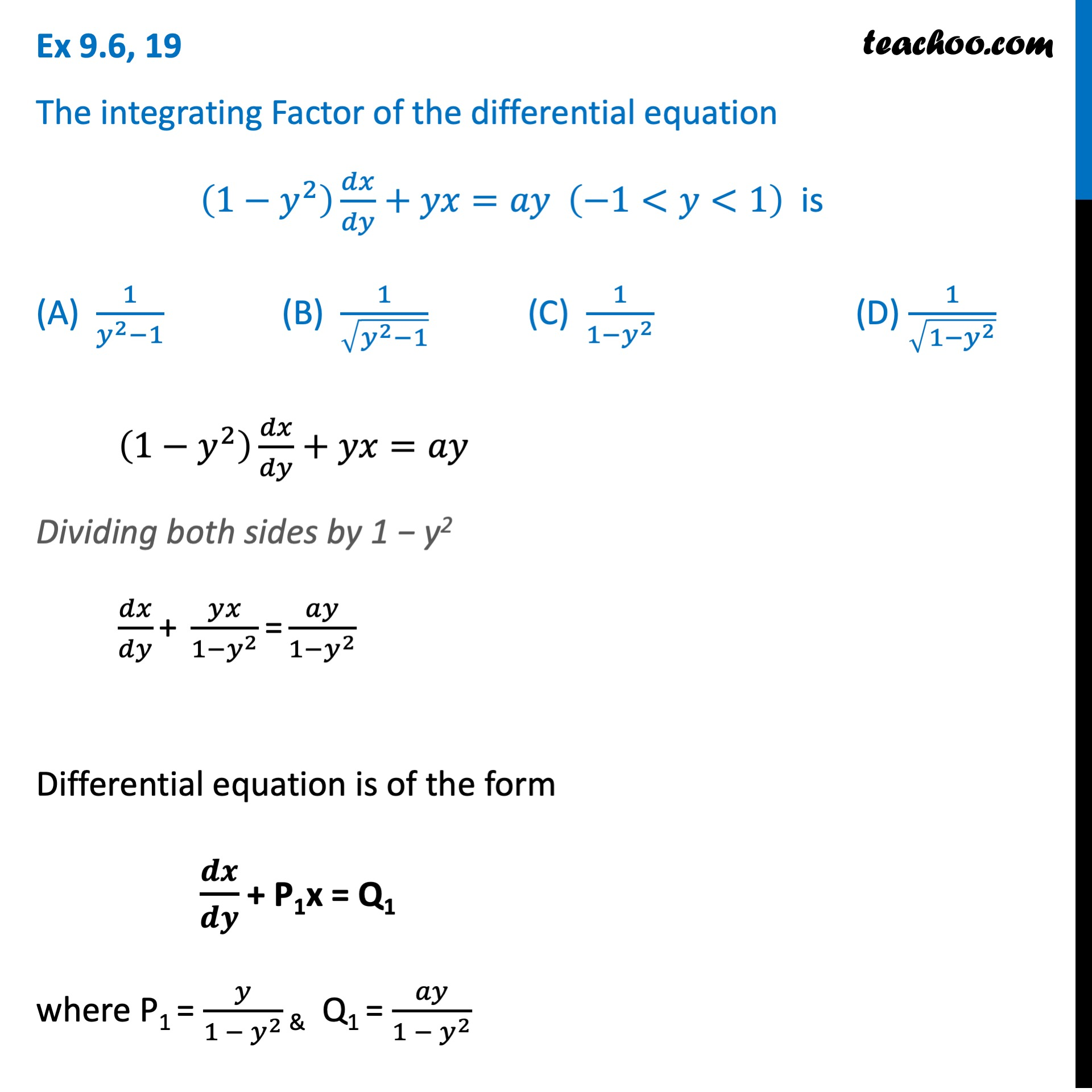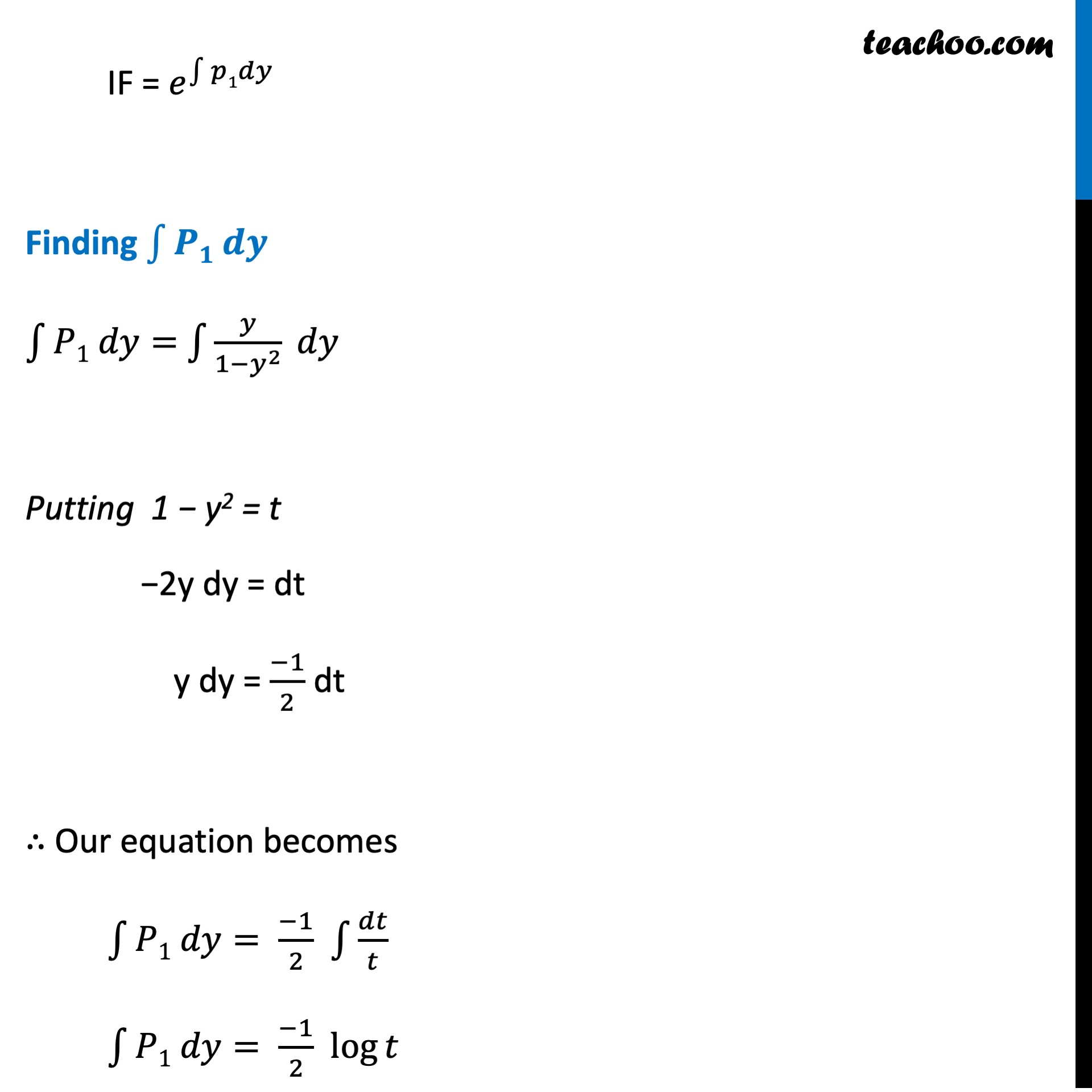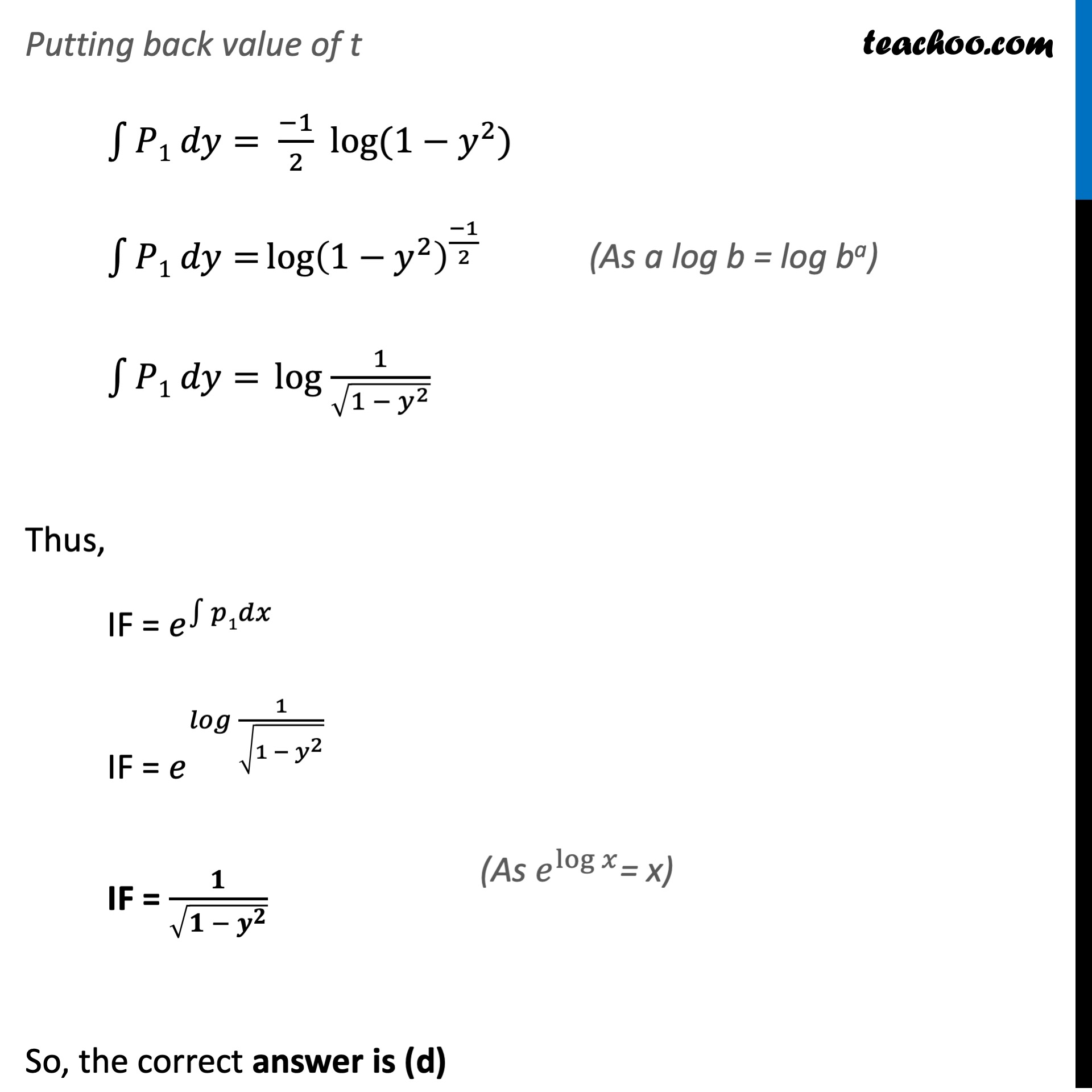Ex 9.6

Chapter 9 Class 12 Differential Equations
Serial order wiseGet live Maths 1-on-1 Classs - Class 6 to 12

### Transcript

Ex 9.6, 19 The integrating Factor of the differential equation (1−𝑦^2 ) 𝑑𝑥/𝑑𝑦+𝑦𝑥=𝑎𝑦 (−1<𝑦<1) is (A) 1/(𝑦^2−1) (B) 1/√(𝑦^2−1) (C) 1/(1−𝑦^2 ) (D) 1/√(1−𝑦^2 ) (1−𝑦^2 ) 𝑑𝑥/𝑑𝑦+𝑦𝑥=𝑎𝑦 Dividing both sides by 1 − y2 𝑑𝑥/𝑑𝑦 + 𝑦𝑥/(1−𝑦^2 ) = 𝑎𝑦/(1−𝑦^2 ) Differential equation is of the form 𝒅𝒙/𝒅𝒚 + P1x = Q1 where P1 = 𝑦/(1 − 𝑦^2 ) & Q1 = 𝑎𝑦/(1 − 𝑦^2 ) IF = 𝑒^∫1▒𝑝1𝑑𝑦 Finding ∫1▒〖𝑷𝟏 𝒅𝒚〗 ∫1▒〖𝑃1 𝑑𝑦=〗 ∫1▒〖𝑦/(1−𝑦^2 ) 𝑑𝑦 〗 Putting 1 − y2 = t −2y dy = dt y dy = (−1)/2 dt ∴ Our equation becomes ∫1▒〖𝑃1 𝑑𝑦= (−1)/2 〗 ∫1▒〖𝑑𝑡/𝑡 〗 ∫1▒〖𝑃1 𝑑𝑦= (−1)/2 〗 log⁡𝑡 Putting back value of t ∫1▒〖𝑃1 𝑑𝑦= (−1)/2 〗 log⁡(1−𝑦^2) ∫1▒〖𝑃1 𝑑𝑦=〗 〖log⁡(1−𝑦^2 )〗^((−1)/2) ∫1▒〖𝑃1 𝑑𝑦= 〗 log 1/√(1 − 𝑦^2 ) Thus, IF = 𝑒^∫1▒𝑝1𝑑𝑥 IF = 𝑒^(𝑙𝑜𝑔 1/√(1 − 𝑦^2 )) IF = 𝟏/√(𝟏 − 𝒚^𝟐 ) So, the correct answer is (d)